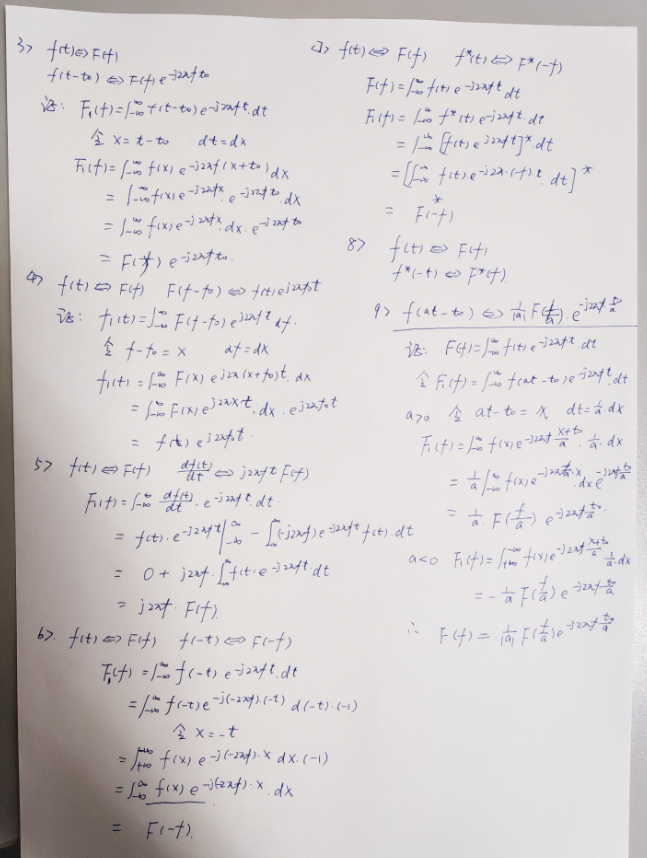• 傅里叶积分变换是实自变量复函数沿复平面实轴无穷区间上的主值积分,可转化为复变函数的环路积分,并利用留数计算来完成,我们导出的实自变量复函数沿复平面实轴无穷区间上的主值积分公式,可用于逆傅里叶积分变换的...
• 在工程技术和科学研究的许多领域,傅里叶积分变换极为重要,但...研究发现,逆傅里叶积分变换可以变换成沿复平面虚轴上的无穷区间主值积分,由此,导出一个逆傅里叶积分变换的计算公式,可用来快速完成逆傅里叶积分变换计算。
• 1、一维傅里叶变换 1.1 一维连续傅里叶变换变换： F(ω)=∫−∞∞f(t)⋅e−iωtdt F(\omega) = \int_{-\infty}^{\infty}f(t)\cdot e^{-i\omega t}dt F(ω)=∫−∞∞​f(t)⋅e−iωtdt 逆变换： f(t)=∫−∞∞...
1、一维傅里叶变换
1.1 一维连续傅里叶变换

正变换：

$F(\omega) = \int_{-\infty}^{\infty}f(t)\cdot e^{-i\omega t}dt$

逆变换：

$f(t) = \int_{-\infty}^{\infty}F(\omega)\cdot e^{i\omega t}d\omega$
1.2 一维离散傅里叶变换

正变换：

$F(u) = \sum_{x=0}^{N-1}f(x)\cdot e^{-i\frac{2\pi}{N}xu} \\ u = 0,1,2, ... , N-1$

逆变换：

$f(x) = \frac{1}{N}\sum_{u=0}^{N-1}F(u)\cdot e^{i\frac{2\pi}{N}xu}\\x = 0,1,2, ... , N-1$
2、二维傅里叶变换
2.1 二维连续傅里叶变换

正变换
$F(u,v)=\int_{-\infty}^{\infty}\int_{-\infty}^{\infty}f(x,y)e^{-j2\pi(ux+vy)}dxdy$

逆变换
$f(x,y)=\int_{-\infty}^{\infty}\int_{-\infty}^{\infty}F(u,v)e^{j2\pi(ux+vy)}dudv$

2.2 二维离散傅里叶变换
令f(x,y)表示一幅大小为MXN像素的数字图像，其中,x=0,1,2,…,M-1, y=0,1,2,…,N-1,由F(u,v)表示的f(x,y)的二维离散傅里叶变换(DFT)由下式给出：
$F(u,v) = \sum_{x=0}^{M-1}\sum_{y=0}^{N-1}f(x,y)e^{-j2\pi(\frac{ux}{M}+\frac{vy}{N})}\\u,v = 0, 1, 2, ... , N-1$
式子当中，u也是属于0到M-1,v属于0到N-1。频率域就是属于u,v作为频率变量，由F(u,v)构成的坐标系，这块MXN的区域我们通常称为频率矩形，很明显频率矩形的大小和输入图像的大小相同。
有傅里叶变换，当然就有傅里叶反变换(IDFT):
$f(x,y) = \frac{1}{MN}\sum_{u=0}^{M-1}\sum_{v=0}^{N-1}F(u,v)e^{j2\pi(\frac{ux}{M}+\frac{vy}{N})}\\ x,y = 0, 1, 2, ... , N-1$
clc,clear;
a = [1 2 3 5 5 ; 4 7 9 5 4;1 4 6 7 5;5 4 3 7 1;8 7 5 1 3];%a矩阵取5*5
b = [1 5 4; 3 6 8; 1 5 7]; %b矩阵如多数模板一样取3*3
c = conv2(a,b)
d = conv2(a,b,'same')

a(7,7) = 0;
b(7,7) = 0;
e = ifft2(fft2(a).*fft2(b)) % .* 对应元素相乘

%
c =
1     7    17    28    42    45    20
7    39    89   127   134   110    56
14    61   151   212   229   177    87
12    74   165   226   245   174    72
24    98   178   190   179   155    55
29    98   179   139   112    80    31
8    47    96    75    43    22    21
%

%
d =

39    89   127   134   110
61   151   212   229   177
74   165   226   245   174
98   178   190   179   155
98   179   139   112    80
%

%
e =

1.0000    7.0000   17.0000   28.0000   42.0000   45.0000   20.0000
7.0000   39.0000   89.0000  127.0000  134.0000  110.0000   56.0000
14.0000   61.0000  151.0000  212.0000  229.0000  177.0000   87.0000
12.0000   74.0000  165.0000  226.0000  245.0000  174.0000   72.0000
24.0000   98.0000  178.0000  190.0000  179.0000  155.0000   55.0000
29.0000   98.0000  179.0000  139.0000  112.0000   80.0000   31.0000
8.0000   47.0000   96.0000   75.0000   43.0000   22.0000   21.0000
%



展开全文• 傅里叶变换的性质中，重点关注的就是 时移，频移，奇偶虚实性，下面给出了详细的推导。 1、欢迎大家关注我的微信公众号： xiaoshi_IC，小石谈IC； 2、2018年4月建号以来，已陆续完成了PCB系列的培训视频的更新，...
傅里叶变换的性质中，重点关注的就是 时移，频移，奇偶虚实性，下面给出了详细的推导。1、欢迎大家关注我的微信公众号： xiaoshi_IC，小石谈IC；
3、2019年计划，目前在持续更新通信原理，以及verilog数字设计部分（目前针对Xilinx FPGA），主要针对通信系统数字设计，软件无线电设计；
4、未来计划：逐步完成 IC版图，信号完整性，IC设计，IC后端，这些是作者很感兴趣的部分，未来会不定期更新，也期望有爱好者共同交流；
5、期望和定位：做开源的、有价值的微电子，电子类公众分享，坚持至少一周一更（目前比较频繁），专注软件无线电，通信算法和工程实现，数字系统设计，不定期放出视频，与大家交流，共同提高，欢迎大家持久关注，订阅，分享，点赞；
6、B站主页：https://space.bilibili.com/382038930
知乎主页：https://www.zhihu.com/people/shishu8385
CSDN主页：https://blog.csdn.net/shishu8385
GitHub主页：https://github.com/shishu8385


展开全文• 首先，傅里叶分析是指把一个周期或非周期函数展开成一个个三角函数的叠加，如果是对其还没有基本概念的，可以看看傅里叶分析之掐死教程，这篇文章不依赖数学公式却又十分透彻地讲述了傅里叶分析的基本概念，十分值得...
首先，傅里叶分析是指把一个周期或非周期函数展开成一个个三角函数的叠加，如果是对其还没有基本概念的，可以看看傅里叶分析之掐死教程，这篇文章不依赖数学公式却又十分透彻地讲述了傅里叶分析的基本概念，十分值得一读。但如果先深入探讨其中的数学由来，接下来会讲述详细的数学推导。
傅里叶级数
三角函数系的正交性
三角函数系：{1,sinx,cosx,sin2x,cos2x,…,sinnx,cosnx,…}，它由无数个sinnx和cosnx组成，其中n=0,1,2,…。
正交性：
$\int^\pi_{-\pi}\sin nx \cos mx dx = 0$
$\int^\pi_{-\pi}\cos nx \cos mx dx = 0，n\neq m$
$\int^\pi_{-\pi}\sin nx \sin mx dx = 0，n\neq m$
当向量点乘等于0：
$\vec a \cdot \vec b = 0$
则两个向量正交。
拓展到函数中，两个函数相乘，原本点乘的加和变成取积分，则：
$\int^{x_1}_{x_2}f(x) g(x) dx = 0$
称为两个函数正交。
证明其正交性，可以用积化和差公式：
$\int^\pi_{-\pi}\cos nx \cos mx dx = \frac{1}{2}\int^\pi_{-\pi}[cos(n-m)x+cos(n+m)x]dx =0$
其他情况同理。
周期为2π的函数展开
因此当一个函数f(x)周期为2π时，可以展开成
$f(x) = \sum^\infty_{n=0}a_ncosnx + \sum^\infty_{n=0}b_nsinnx = \frac 1 2 a_0 + \sum^\infty_{n=1}({a_ncosnx + b_nsinnx})$
对两边取积分，由于三角函数的正交性
$\int_{-\pi}^\pi f(x)dx = \frac1 2\int_{-\pi}^\pi a_0dx = \pi a_0$
$a_0 = \frac 1 {\pi}\int_{-\pi}^\pi f(x)dx$
原式乘以cos mx 再对两边取积分可得：
$\int^\pi_{-\pi}f(x) \cos mx dx = \sum^\infty_{n=1}\int^\pi_{-\pi} a_ncosnx cosmx dx = \int^\pi_{-\pi} a_ncos^2 nx dx = a_n\pi$
$a_n = \frac 1 \pi \int^\pi_{-\pi}f(x) \cos nx dx$
同理，两边同乘sinmx再取积分可得：
$b_n = \frac 1 \pi \int^\pi_{-\pi}f(x) \sin nx dx$
周期为2L的函数展开
利用换元的方法，令：$x = \frac \pi L t$ ，即$t = \frac L \pi x$，可得：
$f(t) = \frac {a_0} 2 + \sum^\infty_{n=1}({a_ncos \frac{n\pi}{L}t + b_nsin\frac{n\pi}{L}t})$
$a_0 = \frac 1 {L}\int_{-L}^L f(t)dt$
$a_n = \frac 1 L \int^L_{-L}f(x) \cos nx dx$
$b_n = \frac 1 L \int^L_{-L}f(x) \sin nx dx$
在工程中t总是从0开始，周期T=2L，ω = $\frac \pi L = \frac 2\pi T$，此时：
$f(t) = \frac {a_0} 2 + \sum^\infty_{n=1}({a_ncosωt + b_nsinωt})$
$a_0 = \frac 2 {T}\int_{0}^T f(t)dt$
$a_n = \frac 2 T \int^T_{0}f(x) \cos nx dx$
$b_n = \frac 2 T \int^T_{0}f(x) \sin nx dx$
傅里叶级数的复数表达形式
欧拉公式：$e^{iθ} = cosθ + isinθ$
可得：
$cosθ = \frac 1 2 (e^{iθ}+e^{-iθ})$
$sinθ = \frac 1 2 i(e^{iθ}-e^{-iθ})$
把这两条式子代入f(t)的式子中可得：
$f(t) = \frac {a_0} 2 + \sum_{n=1}^\infty \frac {a_n-ib_n} 2 e^{in\omega t}+ \sum_{n=1}^\infty \frac {a_n+ib_n} 2 e^{-in\omega t} = \sum_{n=0}^0 \frac {a_0} 2 e^{in\omega t} + \sum_{n=1}^\infty \frac {a_n-ib_n} 2 e^{in\omega t}+ \sum_{n=-1}^{-\infty} \frac {a_{-n}+ib_{-n}} 2 e^{in\omega t} = \sum_{-\infty}^\infty C_n e^{in\omega t}$
当n = 0时，
$C_n = \frac {a_0} 2 = \frac 1 {T}\int_{0}^T f(t)dt$
当n > 0时，
$C_n = \frac {a_n-ib_n} 2 = \frac 1 2 (\frac 2 T \int_0^T f(t)cos{n\omega t} - i\frac 2 T \int_0^Tf(t)sin{n\omega t}) = \frac 1 T \int_0^T f(t)(cos{n\omega t} - isin{n\omega t}) dt = \frac 1 T \int_0^T f(t) e^{-in\omega t}dt$
当n < 0时，
$C_n = \frac {a_{-n}+ib_{-n}} 2 = \frac 1 T \int_0^T f(t)(cos{n\omega t} - isin{n\omega t}) dt = \frac 1 T \int_0^T f(t) e^{-in\omega t}dt$
因此得出结论，一个周期函数f(t)有f(t)=f(t+T)时：
$f(t) = \sum_{-\infty}^\infty C_n e^{in\omega t}$
$C_n = \frac 1 T \int_0^T f(t) e^{-in\omega t}dt$
傅里叶变换
对于$C_n$来说，它的值是一个复数，而nω是一个离散的值，那么可以在代表nω的轴上一个个特定的点上设一个平面，这个平面是一个复平面，它的长度和方向代表$C_n$：在工程上，横坐标为时间的波形图称为时域表达，而这幅图显示的是在各种不同频率下的值，称为频域表达，也是波形图的频谱，这就是从不同的角度看时间，每一种波形都对应一种频谱。不过很多时候的频谱都不是这种复平面三维的，我们会把$C_n$的幅度即模单独拿出来，就可以表示这个函数在不同频率下的强度了。当T增大时，ω数值减小，nω之间就挨得越近。T趋于无穷时会形成一条连续的曲线：由：
$f(t) = \sum_{-\infty}^\infty C_n e^{in\omega t}$
$C_n = \frac 1 T \int_0^T f(t) e^{-in\omega t}dt$
得到：
$f_T(t) = \sum_{n=-\infty}^{\infty}\frac 1 T \int_{-\frac T 2}^{\frac T 2} f_T(t) e^{-in\omega_0 t}dt e^{inω_0t}$
$f_T(t) = \sum_{n=-\infty}^{\infty}\frac {\Deltaω} {2\pi} \int_{-\frac T 2}^{\frac T 2} f_T(t) e^{-in\omega_0 t}dt e^{inω_0t}$
当$T \rightarrow \infty$：
$f(t) = \frac {1} {2\pi} \int_{-\infty}^{\infty} \int_{-\infty}^{\infty} f(t) e^{-i\omega t}dt e^{inωt}d\omega$
因此我们把中间的公式称为傅里叶变换(FT)：
$F(ω) = \int_{-\infty}^{\infty} f(t) e^{-i\omega t}dt$
通过这个函数可以表示在任何频率的情况下的三角函数的波形的振幅，这通常是一个复数a+bi，其中实数部分代表cos，虚数部分代表sin。而负频率没有现实意义，其振幅为正频率的共轭，只是为了在数学上的计算便利，实际上用$\sqrt{a^2+b^2}$表示其振幅。
外面套的公式称为傅里叶变换的逆变换(IFT)：
$f(t) = \frac {1} {2\pi} \int_{-\infty}^{\infty} F(ω) e^{iωt}d\omega$
傅里叶变换的所有内容讲解完毕了，傅里叶变换其实是一种特殊的拉普拉斯变换(s=iω)，遵循拉普拉斯变换的所有性质。


展开全文经验分享
• 离散傅里叶变换公式推导 先抛变换公式： Fm=∑n=0N−1fne−2πimn/N↔fn=1N∑m=0N−1Fme2πimn/N F_m=\sum_{n=0}^{N-1}f_ne^{-2\pi imn/N}\leftrightarrow f_n=\frac{1}{N}\sum_{m=0}^{N-1}F_me^{2\pi imn/N} Fm​=n...
离散傅里叶变换公式推导
先抛变换公式：
$F_m=\sum_{n=0}^{N-1}f_ne^{-2\pi imn/N}\leftrightarrow f_n=\frac{1}{N}\sum_{m=0}^{N-1}F_me^{2\pi imn/N}$
式中的N是数据点个数
讲道理一开始完全看不懂公式这么来的，一顿百度后我学到了很多，但就是没学到怎么推公式。好吧只能自己推。
先来看一下DFT的物理意义：（图我网上随便下的）
离散傅里叶变换是把周期性离散信号变换到频域上，大家知道，周期信号变到频域上是离散的。离散就是在个别点$\{x_n\}$有值。我是学物理的，物理里面离散的可以这么表示：
$f(x)=\sum_{n=0}^{N-1}f_n\delta(x-x_n)$
$\delta(x)$是个在$x=0$处无穷大，其余位置为0且全空间积分为1的函数$\int_{-\infty}^{\infty}\delta(x)dx=1$
周期性信号变到频域上，那不就是傅里叶级数吗。自然有公式
\begin{aligned} F_m &= \int_{-T}^{T}\sum_{n=0}^{N-1}f_n\delta(x-x_n)e^{-ixk_m}dx \\&=\sum_{n=0}^{N-1}\int f_n\delta(x-x_n)e^{-ixk_m}dx \\&=\sum_{n=0}^{N-1}f_ne^{-ix_nk_m} \end{aligned}
接下来我们假设$dx,dk$分别是$\{x_n\}$,$\{k_n\}$的间距，那么：
$x_n=ndx,\qquad k_m = mdk$
代入上式：
\begin{aligned} F_m &=\sum_{n=0}^{N-1}f_ne^{-ix_nk_m} \\&=\sum_{n=0}^{N-1}f_ne^{-imndxdk} \end{aligned}
是不是和最上面的式子很接近了？还差最后一步，确定$dxdk$的值。
下面我懒得写了，只说一下做法吧

先写出$F_m$到$f_n$的逆变换，
$f_n = c\sum_{n=0}^{N-1}F_me^{imndxdk}$
$c$是个系数，之后应该能计算出是$1/N$
把上面的$F_m$表达式带进去,就能得到用$f_{n'}$求和表达的$f_n$,这要求$dxdk$满足一定关系，其实就是满足$dxdk = \frac{2\pi}{N}$
最后把公式里的$dxdk$替换就完事了

这个公式推导倒是不难，主要问题是理解不要出现偏差。所谓离散傅里叶变换是把周期离散信号变换到周期离散频谱，这是真的离散信号。一开始我以为是连续信号在某些给定点采样得到的值呢（没有学过信号相关的内容，在计算物理中遇到了这个离散傅里叶变换）。


展开全文• 傅里叶变换的由来及复数下的傅里叶变换公式证明 1、 考虑到一个函数可以展开成一个多项式的和，可惜多项式并不能直观的表示周期函数，由于正余弦函数是周期函数，可以考虑任意一个周期函数能否表示成为一系列正...
• 本文档中包含了常用的傅里叶变换公式，在学习时可供查阅
• ## 傅里叶变换概念及公式推导

万次阅读 多人点赞 2017-10-16 21:03:16
傅里叶变换公式： （w代表频率，t代表时间，e^-iwt为复变函数） 傅里叶变换认为一个周期函数(信号)包含多个频率分量，任意函数（信号）f(t)可通过多个周期函数（基函数）相加而合成。 从物理角度函数
• 该课件很详细地介绍了傅里叶积分公式，傅里叶变换，傅里叶变换的性质，卷积和卷积定理，很值得下载！
•   让·巴普蒂斯·约瑟夫·傅里叶男爵（1768 －1830）猜测任意周期函数都可以写成三角函数之和。 2 分解的思路   假设f(x)f(x)f(x)是周期为T的函数，傅里叶男爵会怎么构造三角函数的和，使之等于f(x)f(x)f(x)？ ...信号处理 数据挖掘 机器学习
• 根据数学分析中傅里叶级数的相关结论和黎曼积分的思想推导一维连续傅里叶变换和其逆变换公式
• 文章目录 傅里叶变换表示形式 如何计算参数 a0，an，bna_0，a_n，b_na0​，an​，bn​？ 什么条件下函数可以展开成傅里叶级数? 对于周期不是 2π2\pi2π的函数呢？ 傅里叶变换 能量谱密度 功率谱密度 参考文献 ...信号处理 fft
• 因为傅里叶变换之类的很常用，时间长了不用总会忘记，所以一次性罗列出来权当总结好了。主要参考《信号与线性系统分析》(吴大正)，也有的部分参考了复变函数。 δ" role="presentation" style="position: ...
• 但是本人一直好奇傅里叶变换的实际含义和公式是怎么联系在一起的，在阅读了若干篇文章之后，下面是自己的理解： 这是来自https://blog.csdn.net/lzzdflg/article/details/78254381的公式。 ...b h
• 作一维傅里叶变换： (1)F(u)=∫−∞∞f(x)e−j2πuxdx=∫−∞∞e−j2πuxdx=δ(u)F(u)=\int_{-\infty}^{\infty}f(x)e^{-j2\pi ux}dx=\int_{-\infty}^{\infty}e^{-j2\pi ux}dx=\delta(u)\tag1F(u)=∫−∞∞​f(x)e−j...
• sinnwx,cosnwx,....）是一组正交函数，傅里叶变换公式来看就是内积，只有f(t)中含有对应w分 量才能有内积不为零，有对应的频谱。可以理解为在f(t)区间的负无穷到正无穷的内积就是f（t）在e（-jwt）上的投影量。...
• 而这神奇的函数变换规律，就来源于傅里叶变换，学习傅里叶变换，让我们透过现象看本质。 下面的图是由不同的正弦波所构成的矩形脉冲，它就像不同的齿轮相互嵌套旋转所形成。 二、完备正交函数集 我们先从空间中的一...经验分享
• 因为傅里叶变换之类的很常用，时间长了不用总会忘记，所以一次性罗列出来权当总结好了。主要参考《信号与线性系统分析》(吴大正)，也有的部分参考了复变函数。 1.δδ-函数相关运算 nn阶导数的尺度变换 δ(n)...
• 其实一直对傅里叶变换一直停留在认识的层面，今天就要好好梳理一下，为什么这么多人要用它来处理信号，它到底有什么魔力。 那我们就行最初提出的傅里叶级数的公式开始推导吧。三角函数
• 欧拉公式傅里叶变换对我们现在生活的影响真的很大呀！欧拉公式将三角函数和实数函数(e为底的指数函数)互转桥梁。 因此可以把三角函数中复杂的三角变换问题转到较直观的代数运算，也能够把高次幂的正余弦函数...卷积 拉普拉斯变换
• 1.为什么按照傅里叶公式做就可以将信号从时域转变到频域？ 2.为什么式中的e^(-jwt)部分会出现一个负号？有什么特定的意义？修改 举报1 条评论分享•邀请回答 按票数排序 17 个回答 匿名...
• 转接第三章，我们知道了... 它的求法其实没有什么可解释的，无非是看看《信号与系统》或者《傅里叶变换与应用》这些课本。为了完整性这里也是重新带着大家推导一遍：以下推导求的公式，并没有什么物理意义，仅仅只是...
• 一、核心公式 时域==>==>==> 频域 X(w)=∫−∞+∞ x(t)e−jwtdtX(w) = \int_{-\infty}^{+\infty} \,x(t){e^{-jwt}}{\rm d}tX(w)=∫−∞+∞​x(t)e−jwtdt 频域==>==>==> 时域 x(t)=12π∫−∞+∞...信号处理 机器学习 深度学习...